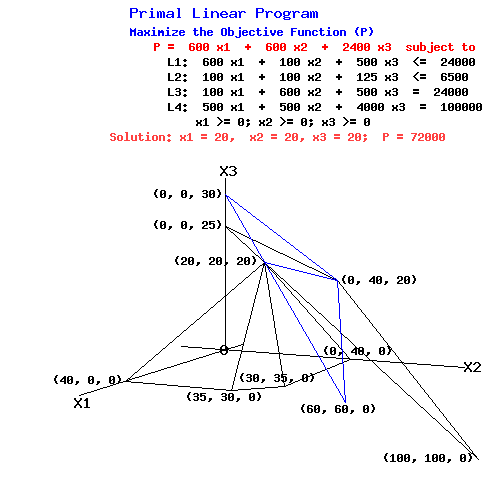Operations Research Problems

by Elmer G. Wiens

Egwald's popular web pages are provided without cost to users.
Please show your support by joining Egwald Web Services as a Facebook Fan:Follow Elmer Wiens on Twitter:1. P.17 of Hillier, Fredrick S. and Lieberman, Gerald J., Operations Research, 2nd edition. San Francisco: Holden-Day, 1974. Wyndor Glass Co. Problem.

max Z = 3 * X1 + 5 * X2

with all the X's non-negative and also with

 X1 <= 4 2*X2 <= 12 3*X1 + 2*X2 <= 18

2. The following problem has 3 variables and 4 <= constraints whose planes intersect in Euclidean space E3 at the optimal vertex – the optimal primal program.

 Primal Linear Program Maximize the Objective Function (P) P = 600 x1 + 600 x2 + 2400 x3 subject to L1: 600.0 x1 + 100.0 x2 + 500.0 x3 <= 24000 L2: 100.0 x1 + 100.0 x2 + 125.0 x3 <= 6500 L3: 100.0 x1 + 600.0 x2 + 500.0 x3 <= 24000 L4: 500.0 x1 + 500.0 x2 + 4000.0 x3 <= 100000       x1 >= 0; x2 >= 0; x3 >= 0

The following three dimensional graphs show how the four restraining planes intersect at the point (20,20,20).

 P-Plane passing through (0, 0, 30), (0, 40, 20), (60, 60, 0), and (20, 20, 20) when P = 72000 L1-plane passing through (40, 0, 0), (35, 30, 0), and (20, 20, 20) L2-plane passing through (35, 30, 0), (30, 35, 0), and (20, 20, 20) L3-plane passing through (30, 35, 0), (0, 40, 0), and (20, 20, 20) L4-plane passing through(0, 0, 25), (0, 40, 20), (100,100, 0), and (20, 20, 20) P-plane & L4-plane intersect along the line passing through (20, 20, 20) and (0, 40, 20) With P = 72000, the P-plane intersects the primal feasible set only at the point (20, 20, 20).The online linear programming solver displays the optimal programs as seen in the next results table.

The L.P. Input Tableau
X1X2X3
Z006006002400
Z124000-600-100-500
Z26500-100-100-125
Z324000-100-600-500
Z4100000-500-500-4000
The L.P. Output Tableau
Y1Y3Y4
Z72000-0.52-0.52-0.47
X120-000
Y200.150.15-0.01
X2200-00
X32000-0

3. Solve the zero sum two person game on P.114 of Intriligator, Michael D., Mathematical Optimization and Economic Theory. Englewood Cliffs, N.J.: Prentice Hall, 1971.

The payoff matrix is:

A =
 6 -2 3 -4 5 4

4. Solve the zero sum two person game on P.761 of Chiang, Alpha C., Fundamental Methods of Mathematical Optimization,. 2nd ed. New York: McGraw-Hill, 1974.

The payoff matrix is:
A =
 10 -1 3 7 2 4 1 0

5. The linear programming problem on P. 152 of Loomba, N. Paul. Linear Programming: An Introductory Analysis. New York: McGraw-Hill, 1964, exhibits degeneracy. Try solving it by hand with the primal simplex method, and then check your answer with the online l.p. solver.

 max P = 22 x1 + 30 x2 + 25 x3 2 x1 + 2 x2 + 0 x3 <= 100 2 x1 + 1 x2 + 1 x3 <= 100 1 x1 + 2 x2 + 2 x3 <= 100 x1 >= 0, x2 >= 0, x3 >= 0

6. Look through the constructed example of "cycling" on P. 190 of Hadley, G. Linear Programming. Reading, Mass: Addison-Wesley, 1962. Then use the dual simplex method to solve the problem.

 max P = .75 x1 - 20 x2 + 0.5 x3 - 6 x4 .25 x1 - 8 x2 - 1 x3 + 9 x4 <= 0 0.5 x1 - 12 x2 - 0.5 x3 + 3 x4 <= 0 0 x1 + 0 x2 + 1 x3 + 0 x4 <= 1 x1 >= 0, x2 >= 0, x3 >= 0, x4 >= 0

7. Dorfman, Robert, Paul Samuelson, and Robert Solow. Linear Programming and Economic Analysis. New York: McGraw-Hill, 1958. In this classical treatise, the authors claim that "much of standard economic analysis is linear programming." They provide the example (85-92) of a firm with 4 production process that convert a limited quantity of raw materials into a product. The variables, x1, x2, x3 , and x4, represent the level of each process's activity. The objective function, P, represents each production process's profitability. Thus "1 unit" of production process 1 generates \$60 of profit from 100 tons of raw materials. The coefficients of the technology matrix, A, capture the efficiency of each production process. The first column of A means: (in a given week) one unit of production process 1 can process 100 tons of raw materials using 7% of input 1 (stills) and 3% of input 2 (retorts).

 max P = 60 x1 + 60 x2 + 60 x3 + 60 x4 100 x1 + 100 x2 + 100 x3 + 100 x4 <= 1,500 7 x1 + 5 x2 + 3 x3 + 2 x4 <= 100 3 x1 + 5 x2 + 10 x3 + 15 x4 <= 100 x1 >= 0, x2 >= 0, x3 >= 0, x4 >= 0

The three constraints represent the availability of inputs: raw materials (1,500 tons), stills (100%), and retorts (100%).

Back to operations research page.Copyright © Elmer G. Wiens:   Egwald Web ServicesAll Rights Reserved.    Inquiries